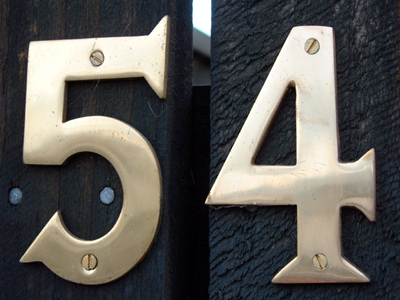54 is the next number of the sequence 18, 27, 36, 45.

# Number Sequences 3

This Math quiz is called 'Number Sequences 3' and it has been written by teachers to help you if you are studying the subject at elementary school. Playing educational quizzes is an enjoyable way to learn if you are in the 3rd, 4th or 5th grade - aged 8 to 11.

It costs only \$12.50 per month to play this quiz and over 3,500 others that help you with your school work. You can subscribe on the page at Join Us

Number sequences involve finding a pattern in order to ascertain what the next number will be. Some patterns are straightforward, such as add 10, but others, like ones involving decimal numbers or fractions, can seem quite difficult to find. But once you know how, sequences with decimal numbers and fractions are just as easy to spot patterns in as ones made of whole numbers. It just takes a bit of practice.

1.
Can you find the rule of this sequence? 14, 21, 28, 35
The numbers increase in 6s
The numbers increase in 7s
The numbers increase in 5s
The numbers increase in 9s
All of the numbers in this sequence are found in the 7 times table
2.
Can you find the rule of this sequence? 6, 12, 18, 24, 30
The numbers increase in 6s
The numbers increase in 3s
The numbers increase in 5s
The numbers increase in 4s
This sequence is the 6 times table
3.
What will be the tenth number of this sequence? 22, 33, 44, 55, 66
108
110
115
121
The numbers are increasing by 11
4.
The difference between two odd numbers is what?
Sometimes odd and sometimes even
Always odd
Always even
It always ends in 0
An odd + an even = an odd so an odd - an odd = an even
5.
What is the next number of the following sequence? 64, 56, 48, 40, 32
35
28
26
24
The numbers are decreasing by 8
6.
The sum of three even numbers will be what?
Even
Odd
It will end in 0
It will end in 5
The sum of 2 even numbers will be even. If you add 2 of the 3 numbers and then add another even it is the same as adding 2 even numbers
7.
Can you find the rule of this sequence? 0, 0.1, 0.2, 0.3, 0.4
The numbers increase in 0.1s
The numbers increase in 0.5s
The numbers increase in 1s
The numbers increase in 0.2s
The sequence would continue: 0.5, 0.6, 0.7, 0.8... etc
8.
The difference between an odd number and an even number is what?
Sometimes odd and sometimes even
Always odd
Always even
It always ends in 0
An odd + an odd = an even so an even - an odd = an odd
9.
The sum of three odd numbers will be what?
Even
Odd
It will end in 0
It will end in 5
The sum of two odd numbers is even but add another odd number and the total will be odd
10.
What is the next number of the following sequence? 18, 27, 36, 45
50
52
54
56
The numbers are increasing by 9
Author:  Amanda Swift